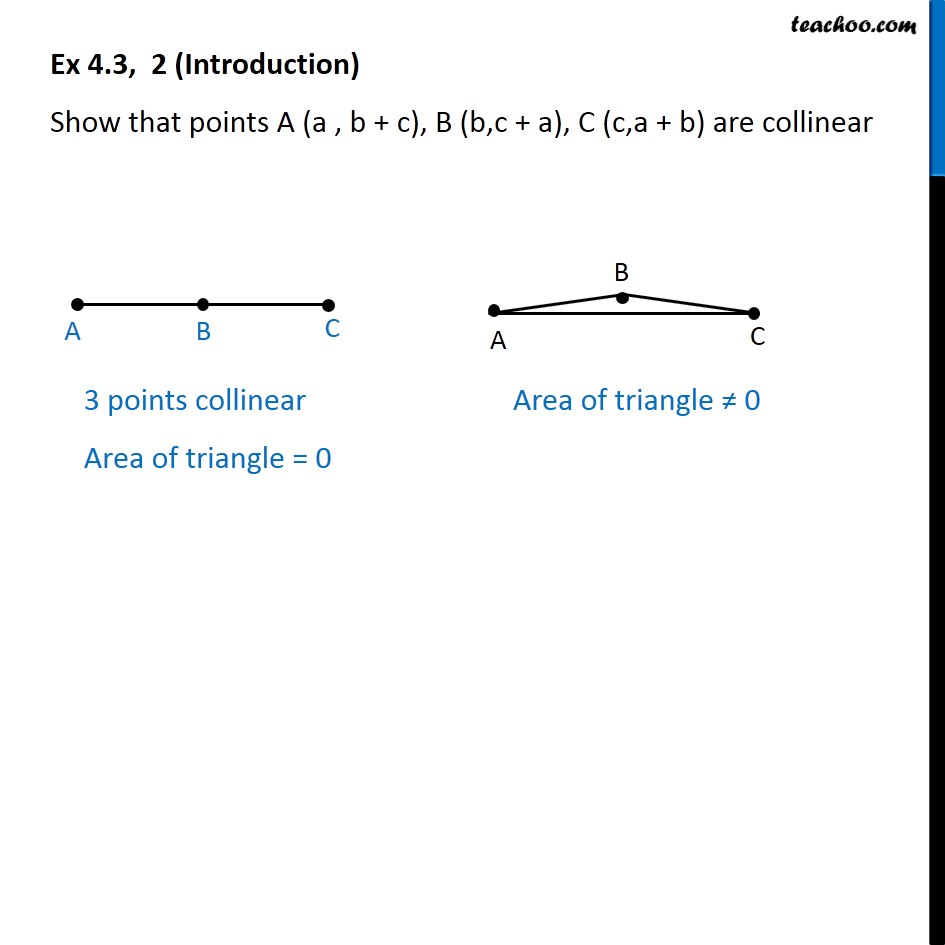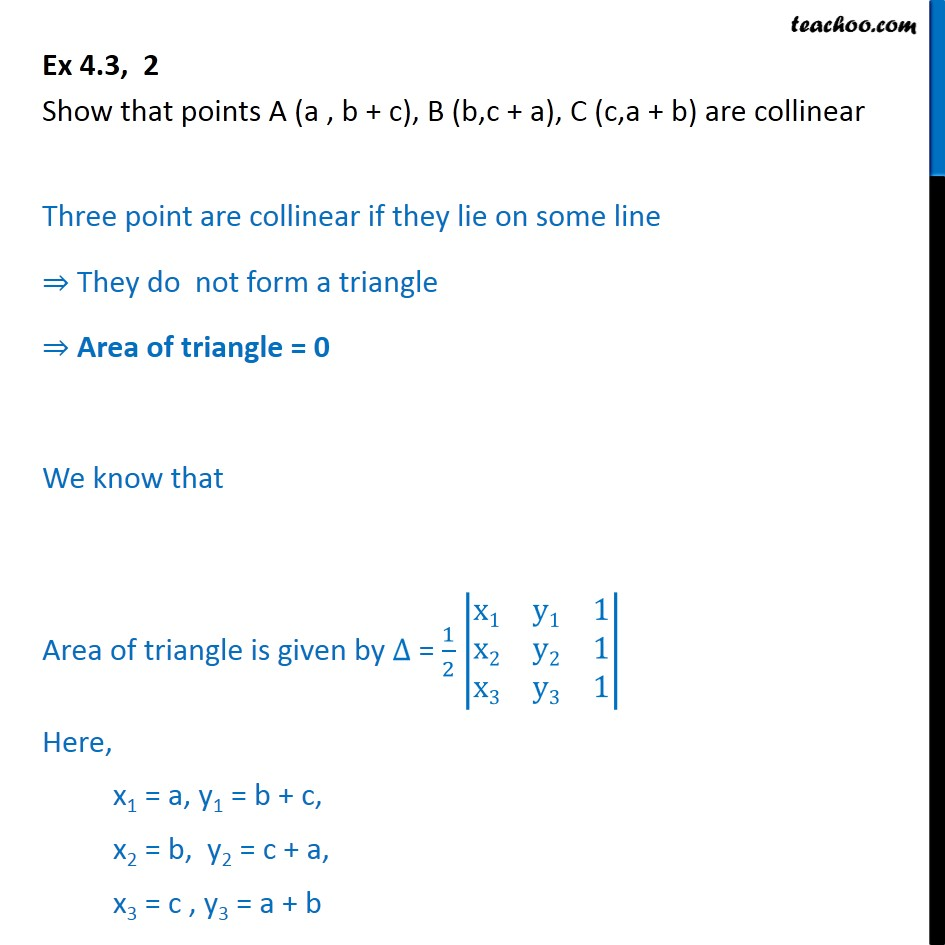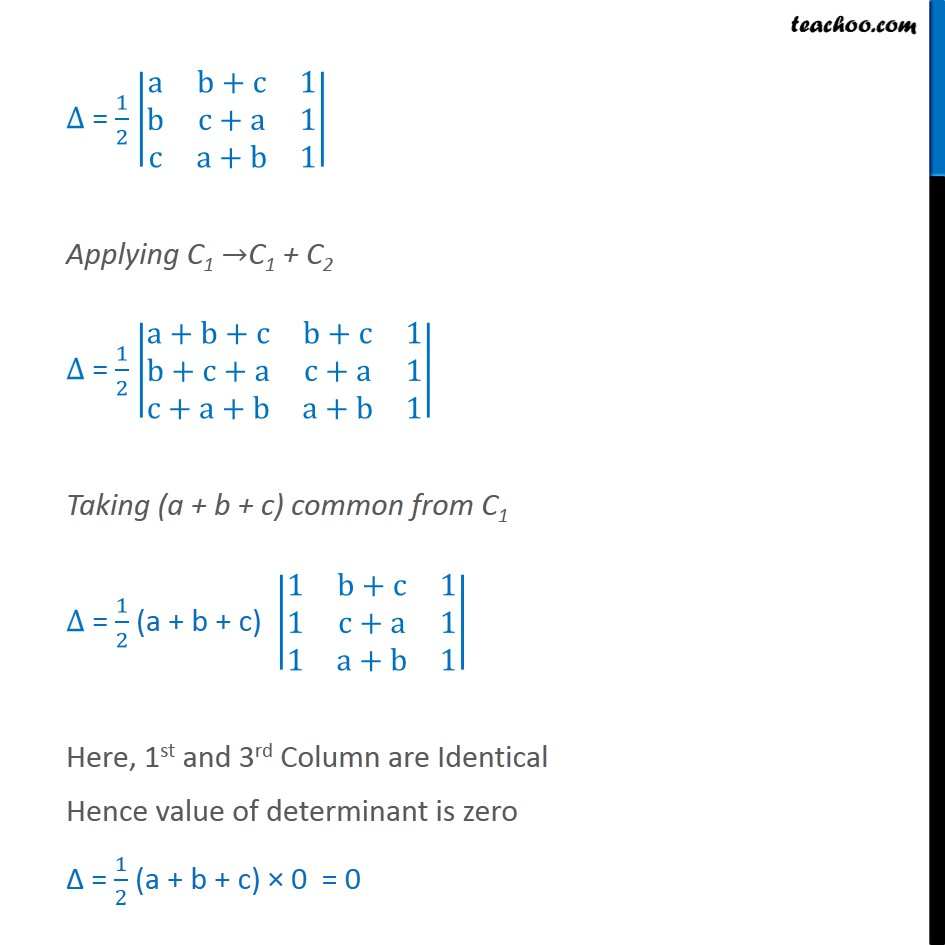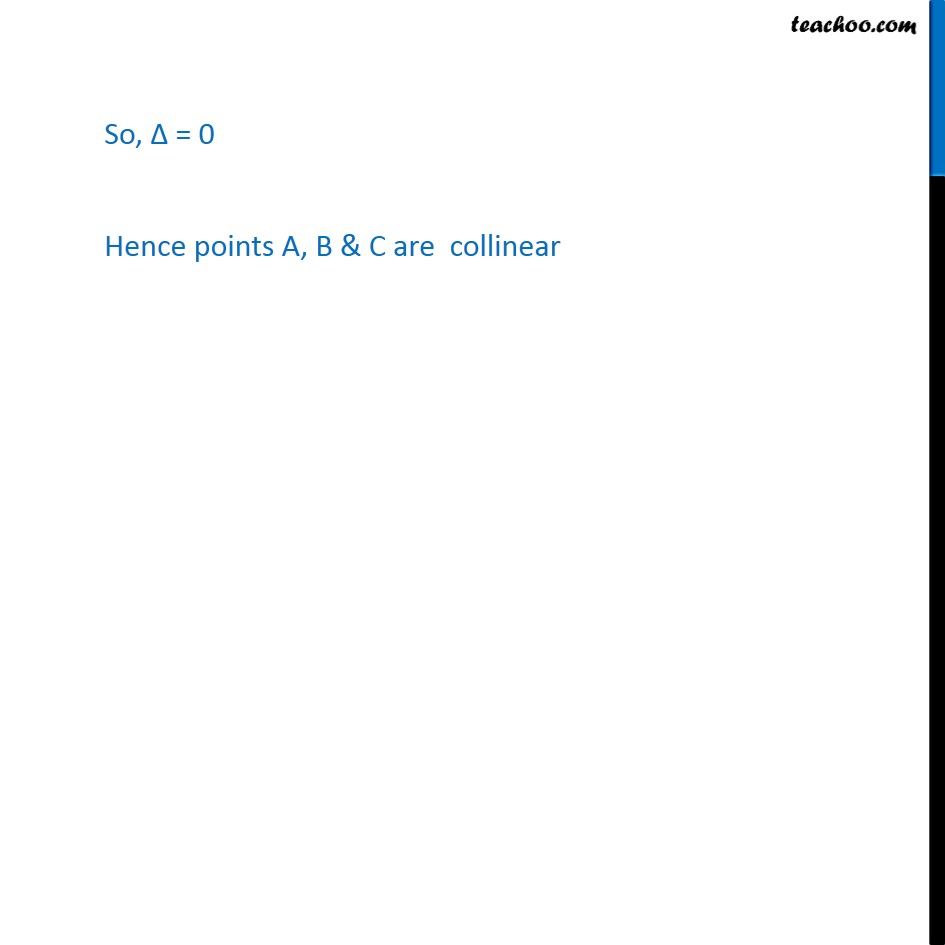1. Class 12
2. Important Question for exams Class 12
3. Chapter 4 Class 12 Determinants

Transcript

Ex 4.3, 2 (Introduction) Show that points A (a , b + c), B (b,c + a), C (c,a + b) are collinear Ex 4.3, 2 Show that points A (a , b + c), B (b,c + a), C (c,a + b) are collinear Three point are collinear if they lie on some line They do not form a triangle Area of triangle = 0 We know that Area of triangle is given by = 1 2 x1 y1 1 x2 y2 1 x3 y3 1 Here, x1 = a, y1 = b + c, x2 = b, y2 = c + a, x3 = c , y3 = a + b = 1 2 a b+c 1 b c+a 1 c a+b 1 Applying C1 C1 + C2 = 1 2 a+b+c b+c 1 b+c+a c+a 1 c+a+b a+b 1 Taking (a + b + c) common from C1 = 1 2 (a + b + c) 1 b+c 1 1 c+a 1 1 a+b 1 Here, 1st and 3rd Column are Identical Hence value of determinant is zero = 1 2 (a + b + c) 0 = 0 So, = 0 Hence points A, B & C are collinear

Chapter 4 Class 12 Determinants

Class 12
Important Question for exams Class 12